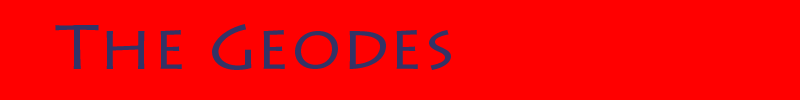### Math Book and Study Sheets

Mathematics is fundamental to knowledge and is critical to success in the modern world. Once you learn advanced mathematics you can learn any other field of study. Do yourself and your children a favor, and study mathematics. It will not only help your career it will help your brain.

Mathematical Methods - Basic Math is designed to provide a thorough understanding of mathematical concepts, definitions, rules, and problem solving techniques, so everyone can succeed at mastering mathematics. The PDF version is free Mathematical Methods - Basic Math. The book can be purchased at Amazon.com.

The following study sheets are free:

Algebra Equations and Rules

Arithmetic Tables - addition, subtraction, multiplication and division Arithmetic Tables

Complex Number Rules

Fractions Rules

Geometry Formulas

Greek Alphabet

Linear Algebra Study Guide

Mathematics Dictionary

Series, Sequences and Summation Rules

Trigonometric Functions and the Unit Circle

Trigonometric Identities and Formulas